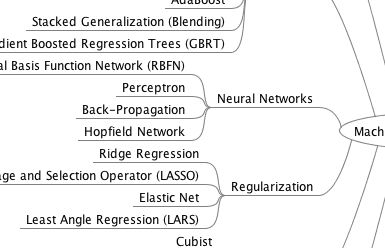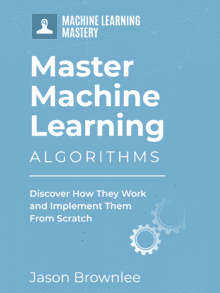# 6 Questions To Understand Any Machine Learning Algorithm

There are a lot of machine learning algorithms and each algorithm is an island of research.

You have to choose the level of detail that you study machine learning algorithms. There is a sweet spot if you are a developer interested in applied predictive modeling.

This post describes that sweet spot and gives you a template that you can use to quickly understand any machine learning algorithm.

Kick-start your project with my new book Master Machine Learning Algorithms, including step-by-step tutorials and the Excel Spreadsheet files for all examples.Sweet Spot For Understanding Machine Learning Algorithms
Photo by dmums, some rights reserved.

Let’s get started.

## What You Need To Know About a Machine Learning Algorithm?

What do you need to know about a machine learning algorithm to be able to use it well on a classification or prediction problem?

I won’t argue that the more that you know about how and why a particular algorithm works, the better you can wield it. But I do believe that there is a point of diminishing returns where you can stop, use what you know to be effective and dive deeper into the theory and research on an algorithm if and only if you need to know more in order to get better results.

Let’s take a look at the 6 questions that will reveal how a machine learning algorithms and how to best use it.

## Get your FREE Algorithms Mind MapSample of the handy machine learning algorithms mind map.

I've created a handy mind map of 60+ algorithms organized by type.

There are 6 questions that you can ask to get to the heart of any machine learning algorithm:

1. How do you refer to the technique (e.g. what name)?
2. How do you represent a learned model (e.g. what coefficients)?
3. How to you learn a model (e.g. the optimization process from data to the representation)?
4. How do you make predictions from a learned model (e.g. apply the model to new data)?
5. How do you best prepare your data for the modeling with the technique (e.g. assumptions)?
6. How do you get more information on the technique (e.g. where to look)?

You will note that I have phrased all of these questions as How-To. I did this intentionally to separate the practical concerns of how from the more theoretical concerns of why. I think knowing why a technique works is less important than knowing how it works, if you are looking to use it as a tool to get results. More on this in the next section.

Let’s take a closer look at each of these questions in turn.

### 1. How Do You Refer To The Technique?

This is obvious but important. You need to know the canonical name of the technique.

You need to be able to recognize the classical name or the name of the method from other fields as well and know that it is the same thing. This also includes the acronym for the algorithm, because sometimes they are less than intuitive.

This will help you sort out the base algorithm from extensions and the family tree of where the algorithm fits and relates to similar algorithms.

### 2. How Do You Represent a Learned Model?

I really like this nuts and bolts question.

This is question often overlooked in textbooks and papers and is perhaps the first question an engineer has when thinking about how a model will actually be used and deployed.

The representation is the numbers and data structure that captures the distinct details learned from data by the learning algorithm to be used by the prediction algorithm. It’s the stuff you save to disk or the database when you finalize your model. It’s the stuff you update when new training data becomes available.

Let’s make this concrete with an example. In the case of linear regression, the representation is the vector of regression coefficients. That’s it. In the case of a decision tree is is the tree itself including the nodes, how they are connected and the variables and cut-off thresholds chosen.

### 3. How Do You Learn a Model?

Given some training data, the algorithm needs to create the model or fill in the model representation. This question is about exactly how that occurs.

Often learning involves estimating parameters from the training data directly in simpler algorithms.

In most other algorithms it involves using the training data as part of a cost or loss function and an optimization algorithm to minimize the function. Simpler linear techniques may use linear algebra to achieve this result, whereas others may use a numerical optimization.

Often the way a machine learning algorithm learns a model is synonymous with the algorithm itself. This is the challenging and often time consuming part of running a machine learning algorithm.

The learning algorithm may be parameterized and it is often a good idea to list common ranges for parameter values or configuration heuristics that may be used as a starting point.

### 4. How Do You Make Predictions With A Model?

Once a model is learned, it is intended to be used to make predictions on new data. Note, we re exclusively talking about predictive modeling machine learning algorithms for classification and regression problems.

This is often the fast and even trivial part of using a machine learning algorithm. Often it is so trivial that it is not even mentioned or discussed in the literature.

It may be trivial because prediction may be as simple as filling in the inputs in an equation and calculating a prediction, or traversing a decision tree to see what leaf-node lights up. In other algorithms, like k-nearest neighbors the prediction algorithm may be the main show (k-NN has no training algorithm other than “store the whole training set”).

### 5. How Do You Best Prepare Data For The Algorithm?

Machine learning algorithms make assumptions.

Even the most relaxed non-parametric methods make assumptions about your training data. It is good or even critical to review these assumptions. Even better is to translate these assumptions into specific data preparation operations that you can perform.

This question flushes out transforms that you could use on your data before modeling, or at the very least gives you pause to think about data transforms to try. What I mean by this is that it is best to treat algorithm requirements and assumptions as suggestions of things to try to get the most out your model rather than hard and fast rules that your data must adhere to.

Just like you cannot know which algorithm will be best for your data before hand, you cannot know the best transforms to apply to your data to get the most from an algorithm. Real data is messy and it is a good idea to try a number of presentations of your data with a number of different algorithms to see what warrants deeper investigation. The requirements and assumptions of machine learning algorithms help to point out presentations of your data to try.

Some algorithms will bubble up as generally better than others on your data problem.

When they do, you need to know where to look to get a deeper understanding of the technique. This can help with further customizing the algorithm for your data and with tuning the parameters of the learning and prediction algorithms.

It is a good idea to collect and list resources that you can reference if and when you need to dive deeper. This may include:

• Journal Articles
• Conference Papers
• Books including textbooks and monographs
• Webpages

I also think it is a good idea to know of more practical references like example tutorials and open source implementations that you can look inside to get a more concrete idea of what is going on.

For more on researching machine learning algorithms, see the post How to Research a Machine Learning Algorithm.

## Summary

In this post you discovered 6 questions that you can ask of a machine learning, that if answered, will give you a very good and practical idea of how it works and how you can use it effectively.

These questions were focused on machine learning algorithms for predictive modeling problems like classification and regression.

These questions, phrased simply are:

1. What are the common names of the algorithm?
2. What representation is used by the model?
3. How does the algorithm learn from training data?
4. How can you make predictions from the model on new data?
5. How you can best prepare your data for the algorithm?

For another post along this theme of defining an algorithm description template see How to Learn a Machine Learning Algorithm.

Do you like this approach? Let me know in the comments.

## Discover How Machine Learning Algorithms Work!#### See How Algorithms Work in Minutes

...with just arithmetic and simple examples

Discover how in my new Ebook:
Master Machine Learning Algorithms

It covers explanations and examples of 10 top algorithms, like:
Linear Regression, k-Nearest Neighbors, Support Vector Machines and much more...

### 2 Responses to 6 Questions To Understand Any Machine Learning Algorithm

1.Mark October 3, 2016 at 11:16 pm #

About things like “which types of problems is this algorithm most suitable for?” and “what are the strengths and weaknesses?”.

Are these less important, or is it better to understand the “how” details you mention first, and then derive the answers to these questions?

•Jason Brownlee October 4, 2016 at 7:23 am #

Hi Mark,

Algorithm suitability is hard. Generally, you can note things like classification/regression as requirements. Beyond that, it’s really an unknowable problem. You have to spot-check and discover suitability problem-by-problem.

Strengths and weaknesses are a great thing to focus on, instead I phrase them as usage heuristics. Same idea.

How it works is key to everything, the lens to understand everything else about the algorithm. The why is usually a bunch of math that few people understand deeply and probably not worth the effort, at least not for a while.